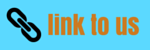# Class 10 electricity Notes : Electric Power

## 12. Electric Power

• Rate of doing work or the rate of consumption of energy is known as POWER
Mathematically,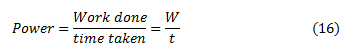• SI unit of power is Watt which is denoted by letter W. The power of 1 Watt is a rate of working of 1 Joule per second. Actually Watt is a small unit, therefore , a bigger unit of electric power called Kilowatt is used for commercial purposes. Also ,
1 kilowatt = 1000 Watts
So,
" the rate at which electric work is done or the rate at which electric energy is consumed is called electric power "
• We will now derive formula for the calculation of electric power.
From equation 14 we know that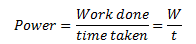Now we know that work done W by current I when it flows for time t under a potential difference V is given by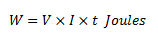Putting this value of work done in equation 16 we get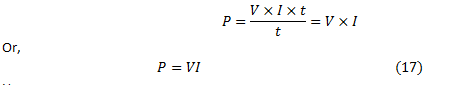Hence,
Electric Power = voltage x current

### 12 (a) Power in terms of I and R

• From equation 17 we know that
$P=VI$
Now from Ohm's law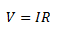Putting above equation in equation 15 we get
$P=I \times R \times I$
Power , $P=I^2 \times R$
• Above formula is used to calculate power when only current and resistance are known to us.

### 12 (b) Power in terms of V and R

• From equation 17 we know that P=VI Now from Ohm's lawOr we have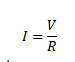• Putting this value of I in equation 15 we get
$P = V \times \frac{V}{R}$
$P = \frac{V^2}{R}$
This formula is used for calculating power when voltage V and resistance R is known to us.
• It is clear from the above equation that power is inversely proportional to the the resistance.
• Thus the resistance of high power devices is smaller then the low power ones. For example , the resistance of a 100 Watt bulb (220 V) is smaller then that of 60 Watt (220 V) bulb.
• We have three formulas for calculating electric power. These are
(1) $P = V \times I$
(2) $P= I^2 \times R$
(3)$P = \frac{V^2}{R}$
You must memorize these formulas as they would be used to solve numerical problems.
• When electrical appliance consumes electrical energy at the rate of 1 Joule per second , its power is said to be 1 Watt.
• Rate at which electric work is done or the rate at which electric energy is consumed , is called electrical power.